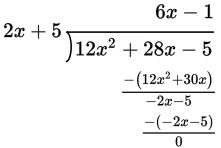# SAT Math Multiple Choice Question 908: Answer and Explanation

### Test Information

Question: 908

8.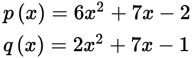Two polynomial functions p(x) and q(x) are defined above. Which of the following polynomial functions is divisible by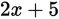?

• A.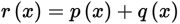• B.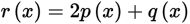• C.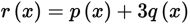• D.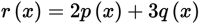Explanation:

C

Difficulty: Hard

Category: Passport to Advanced Math / Exponents

Strategic Advice: Take a moment to think about this question logically before you start multiplying functions by constants and adding functions. Understanding what it means for a polynomial to be divisible by an expression can cut your work in half.

Getting to the Answer: If a polynomial is divisible by an expression, then the remainder when divided will be 0. The only way the remainder can be 0 is if the constant term of the expression divides evenly into the constant term of the polynomial. Based only on the constant terms:

A: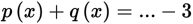is not divisible by 5.

B: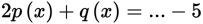is divisible by 5.

C: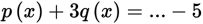is divisible by 5.

D: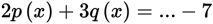is not divisible by 5.

The answer must be B or (C). Try B: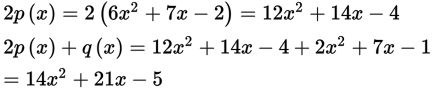Now use long division: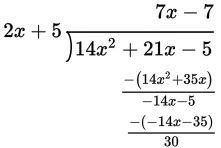The remainder is not 0 (it's 30), so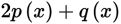is not divisible by 2x + 5. This means (C) must be the correct answer. (For extra practice, work it out using the steps above to confirm that the remainder is indeed 0, but keep in mind that this is not necessary on Test Day.)